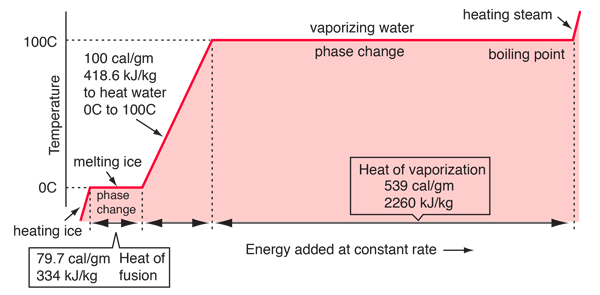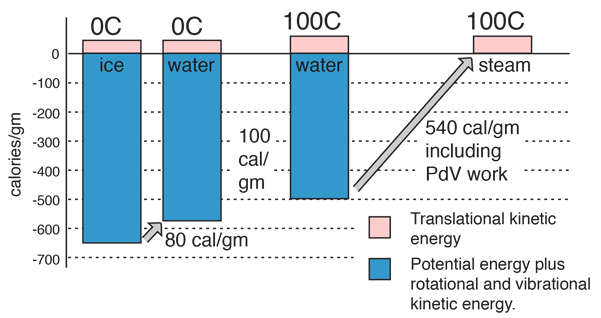# Phase changes

Transitions between solid, liquid, and gaseous phases typically involve large amounts of energy compared to the specific heat. If heat were added at a constant rate to a mass of ice to take it through its phase changes to liquid water and then to steam, the energies required to accomplish the phase changes (called the latent heat of fusion and latent heat of vaporization ) would lead to plateaus in the temperature vs time graph. The graph below presumes that the pressure is one standard atmosphere.Temperature scales Water phase changes Boiling point
 Water
Index

Phase change concepts

 HyperPhysics***** Thermodynamics R Nave
Go Back

# Energy Involved in the Phase Changes of WaterThe data for the vaporization phase change presumes that the pressure is one standard atmosphere.

 Why negative potential energy? Heat of fusion Division of energy Heat of vaporization
 Water
Index

Phase change concepts

 HyperPhysics***** Thermodynamics R Nave
Go Back

# Why is the Potential Energy Negative?

In discussing the energy of the phase changes in water, we found that the potential energy is treated as a negative quantity. An analogy with a mechanical system with gravitational potential energy and kinetic energy might be helpful in understanding the logic of a negative energy quantity. You are always free to choose the zero of potential energy, and it seems logical to choose the zero of potential energy such that a free molecule at rest has zero energy. A bound particle at rest then has negative potential energy.Water phase changes
Index

Phase change concepts

 HyperPhysics***** Thermodynamics R Nave
Go Back

# Details of Heating Water

It is known that 100 calories of energy must be added to raise the temperature of one gram of water from 0° to 100°C. Part of that energy increases the kinetic energy of the molecules, and some adds to the potential energy.The sizes of the blocks which represent the kinetic energy of the molecules at 0°C and 100°C provide a visual illustration of the meaning of temperature and the nature of the absolute or Kelvin temperature scale. From the definition of kinetic temperature, the size of the block is seen to be proportional to temperature, and the ratios of the heights of the KE blocks is the ratio of the temperatures. But the kinetic temperature is inherently the absolute temperature, so that the ratio of the heights of the blocks is 373K/273K . So the absolute temperature is actually proportional to the translational kinetic energy of the molecules, while the Celsius temperatures are just chosen for convenience.

 Water phase changes More detail about energy changes
 Water
Index

Phase change concepts

 HyperPhysics***** Thermodynamics R Nave
Go Back

# Some energy details related to heating water

In the process of heating water from 0 to 100 C, 100 calories of energy must be added. Part of that energy increases the kinetic energy of the molecules, and some adds to the potential energy. To assess the amount added to kinetic energy, the molecular speeds at the two temperatures may be evaluated with the Boltzmann speed distribution.The net gain in kinetic energy is then 16.7 calories/gram when the water is heated from 0 to 100 C. The remainder of the energy goes into weakening the attractive forces between the water molecules. This weakening of the intermolecular forces manifests itself in the reduction of the surface tension of water as it is heated.

In the process of vaporization of water, a large amount of energy must be added to overcome the remaining cohesive forces between the molecules and an additional amount of energy goes into PdV work to expand the gas from its very small liquid volume to the volume occupied by the resulting vapor.If the heat of vaporization of water at 100°C is 539 calories, then subtracting the 41 calorie work component suggests that the actual binding energy of the water molecules at 100°C is 539-41=498 calories.

## Why is the heat of vaporization more at body temperature?

An interesting feature of the process of cooling the human body by evaporation is that the heat extracted by the evaporation of a gram of perspiration from the human skin at body temperature (37°C) is quoted in physiology books as 580 calories/gm rather than the nominal 540 calories/gm at the normal boiling point. The question is, why is it larger at body temperature?

The main part of the answer is that the binding energy of the water molecules is greater at that lower temperature, and it therefore takes more energy to break them apart into the gaseous state. The change in the heat of vaporization can be roughly calculated using what we know from the specific heat of water, 1 calorie/gm °C. It takes 37 calories to heat a gram of water from 0°C to 37°C, but the change in the kinetic energy is much less than that:It was shown above that the kinetic energy of the water molecules only increases by 61.7 - 45 = 16.7 calories/gm when the water is heated from zero to 100°C but we know it takes 100 calories to do that heating. Therefore the contribution to weakening the water bonds is 83.3 calories/gm. Using the result for water at 37°C it is evident that 52.4 calories of additional energy must be supplied at 37°C to vaporize the water.

There is one additional element in modeling the heat of vaporization at body temperature - the PdV work required to expand the water into its gaseous form is slightly less at 37°C. By analogy with the work calculation above, that work is found to be 34.2 calories/gm, 6.8 calories/gm less than at 100°C.

This model then suggests a heat of vaporization at 37°C:

Body temperature heat of vaporization = 539 cal/gm + 52.4 cal/gm - 6.8 cal/gm = 585 cal/gm.

So this simple model agrees fairly well with the quoted 580 cal/gm.

 Water phase changes
 Water
Index

Phase change concepts

 HyperPhysics***** Thermodynamics R Nave
Go Back